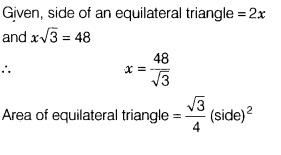# Each side of an equilateral triangle is 2x cm. If x√3 =48, then find its area

Each side of an equilateral triangle is 2x cm. If x√3 =48, then find its area.